Geometry 4-2 Triangle Congruence by SSS and SAS
starstarstarstarstarstarstarstarstarstar
by Scott Phillips
| 21 Questions
The outlined content above was added from outside of Formative.
The outlined content above was added from outside of Formative.1
2
1
10 pts
Solve It! Are the triangles shown congruent?
Yes
No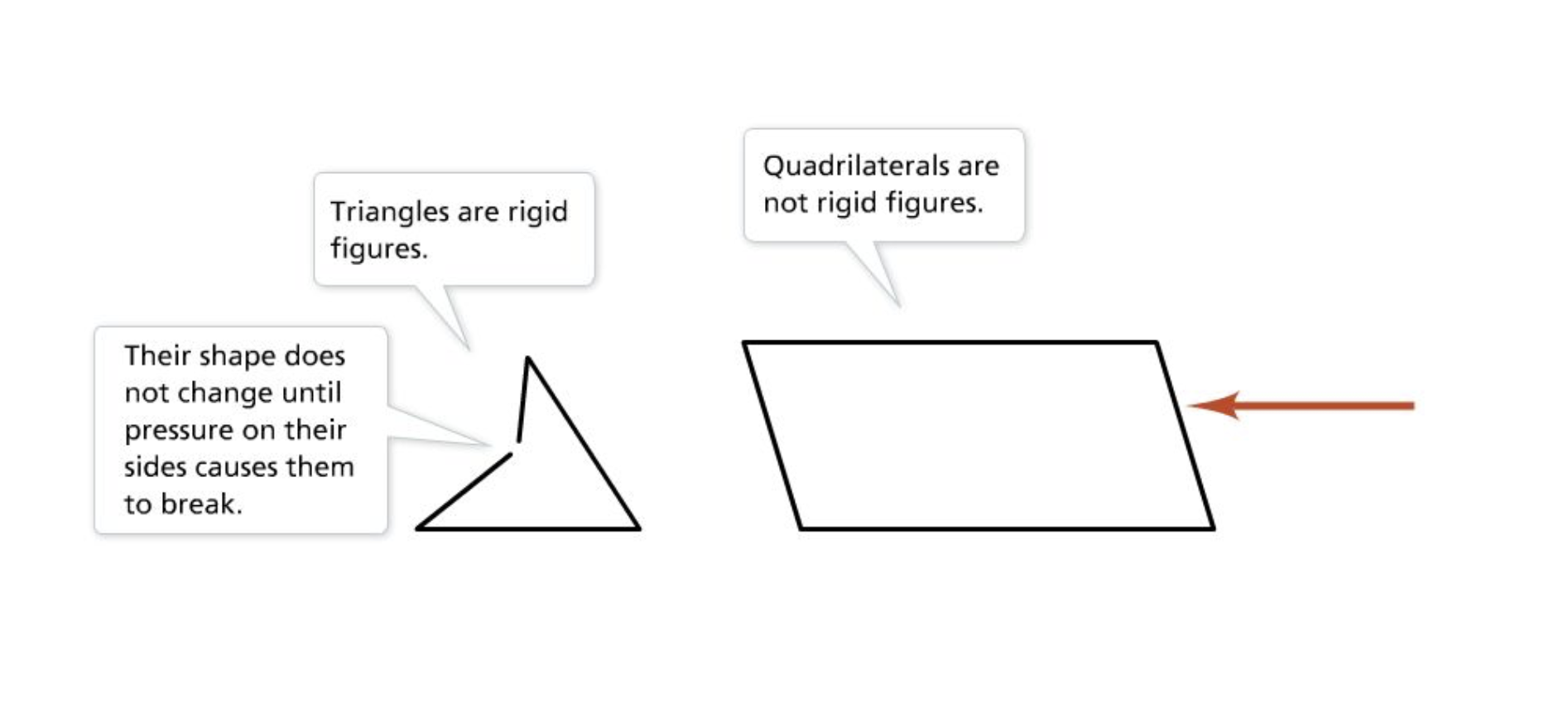The outlined content above was added from outside of Formative.
3
30 pts
Problem 1 Got It? Complete the proof on the canvas.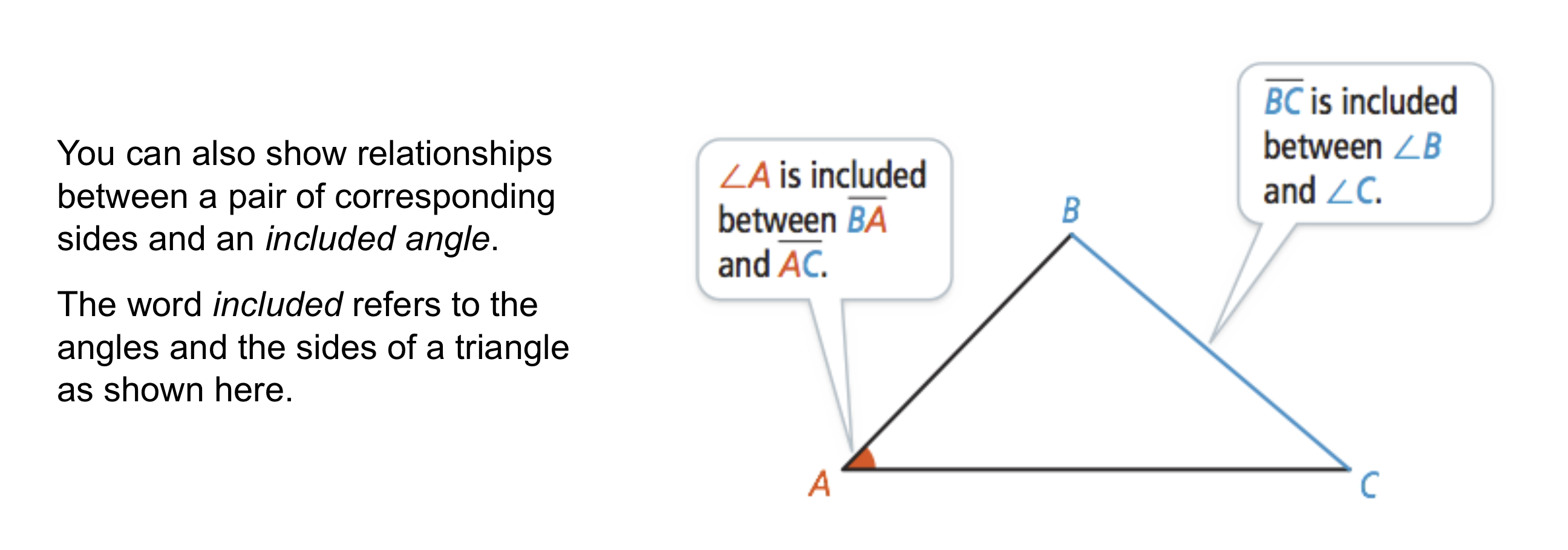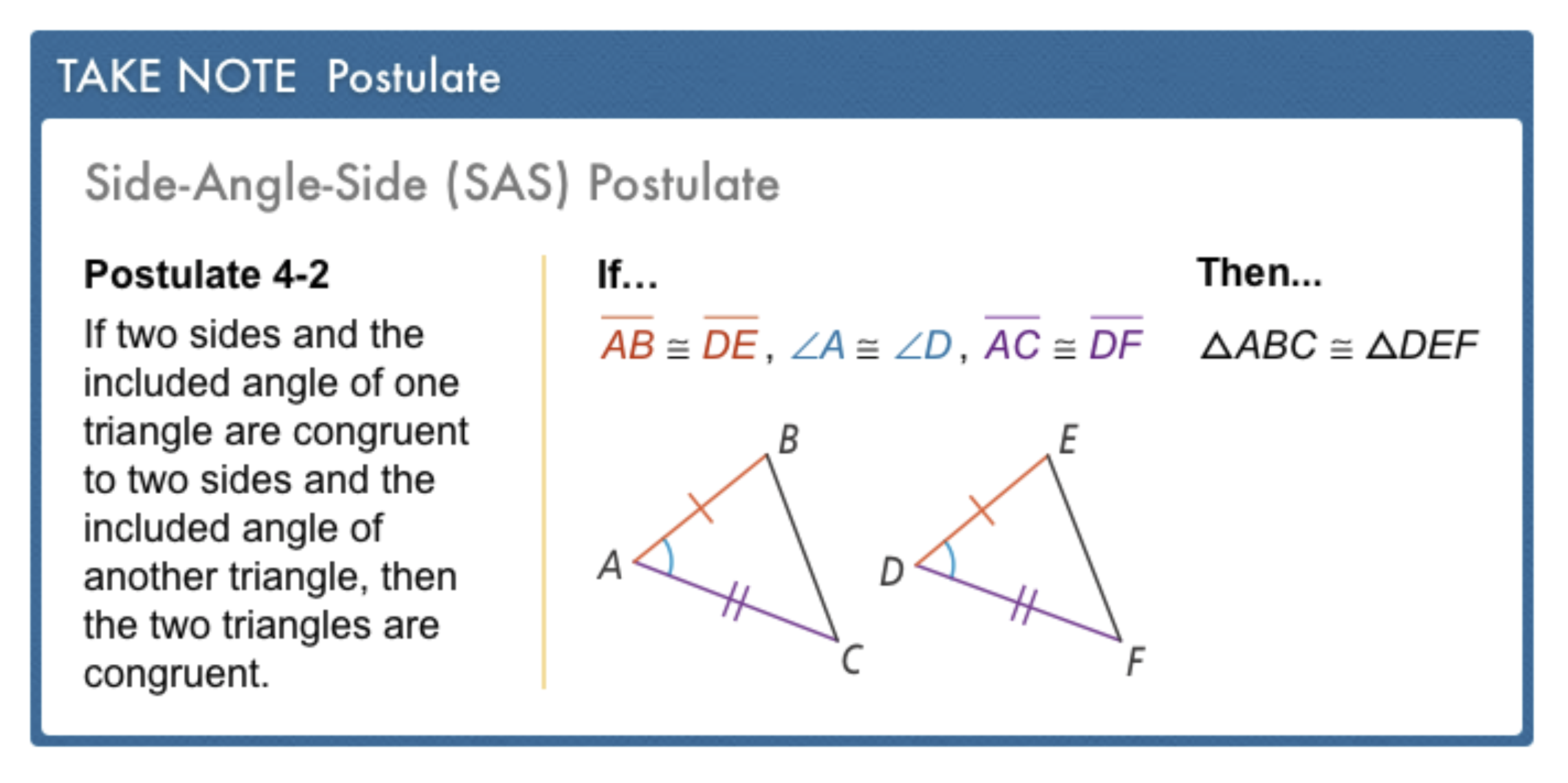The outlined content above was added from outside of Formative.4
4
10 pts
Problem 2 Got It?
A
B
C
DThe outlined content above was added from outside of Formative.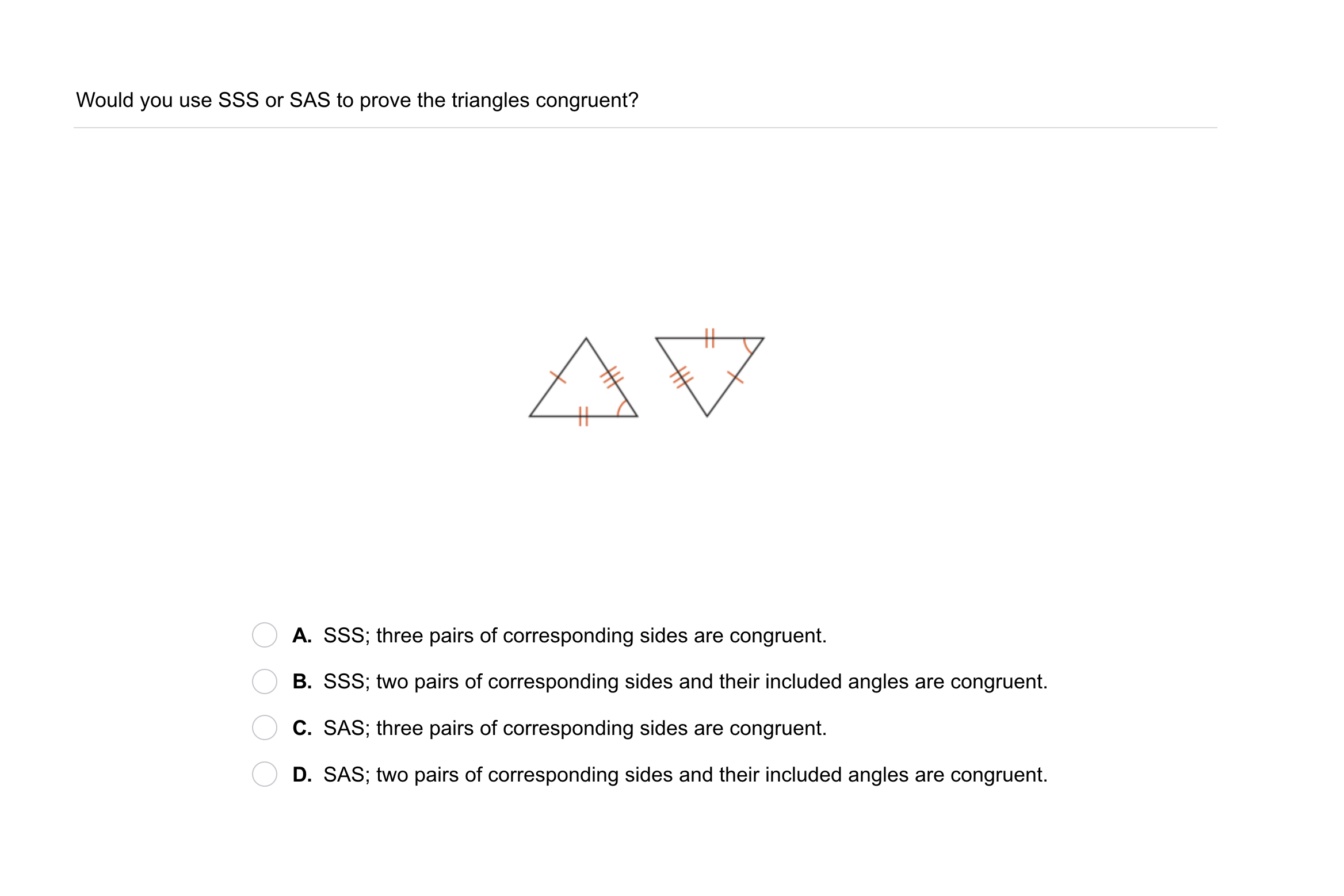5
5
10 pts
Problem 3 Got It?
A
B
C
D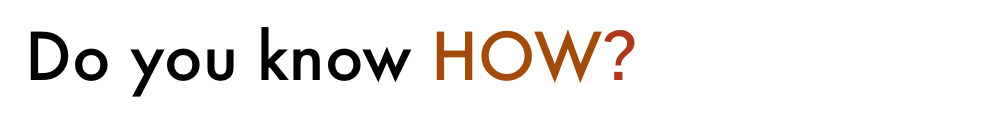6
6
10 pts
A
B
C
D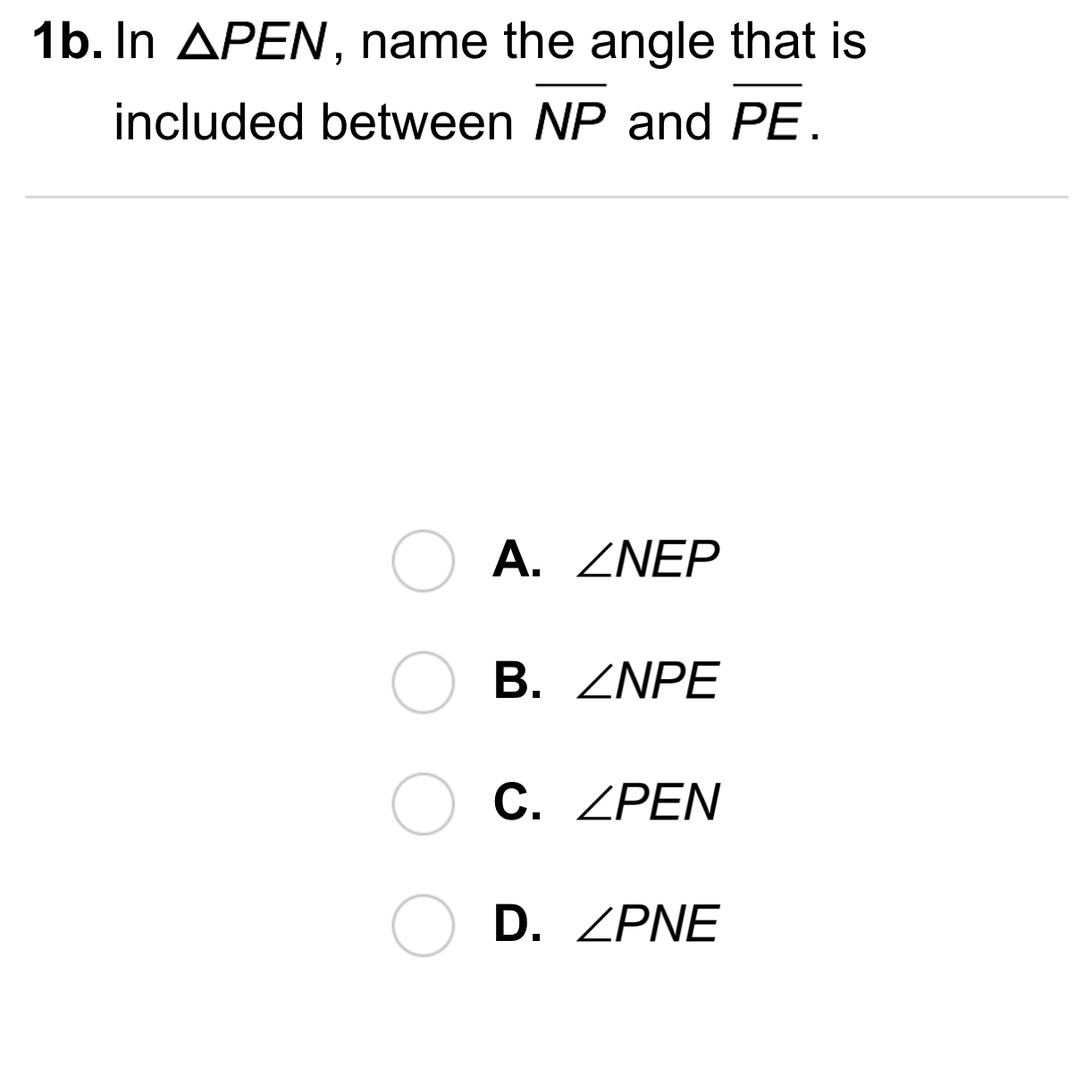7
7
10 pts
A
B
C
D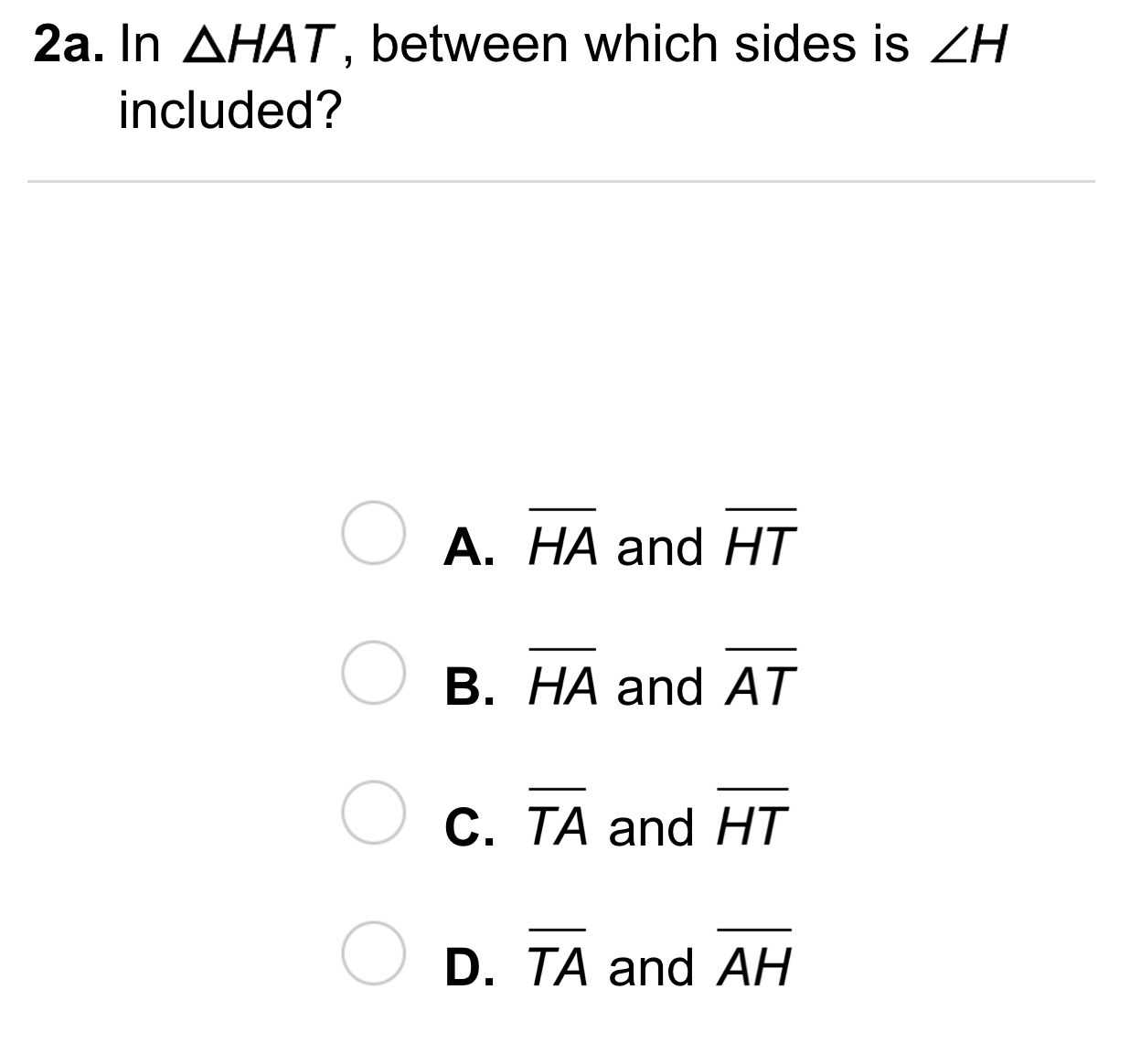8
8
10 pts
A
B
C
D9
9
10 pts
A
B
C
D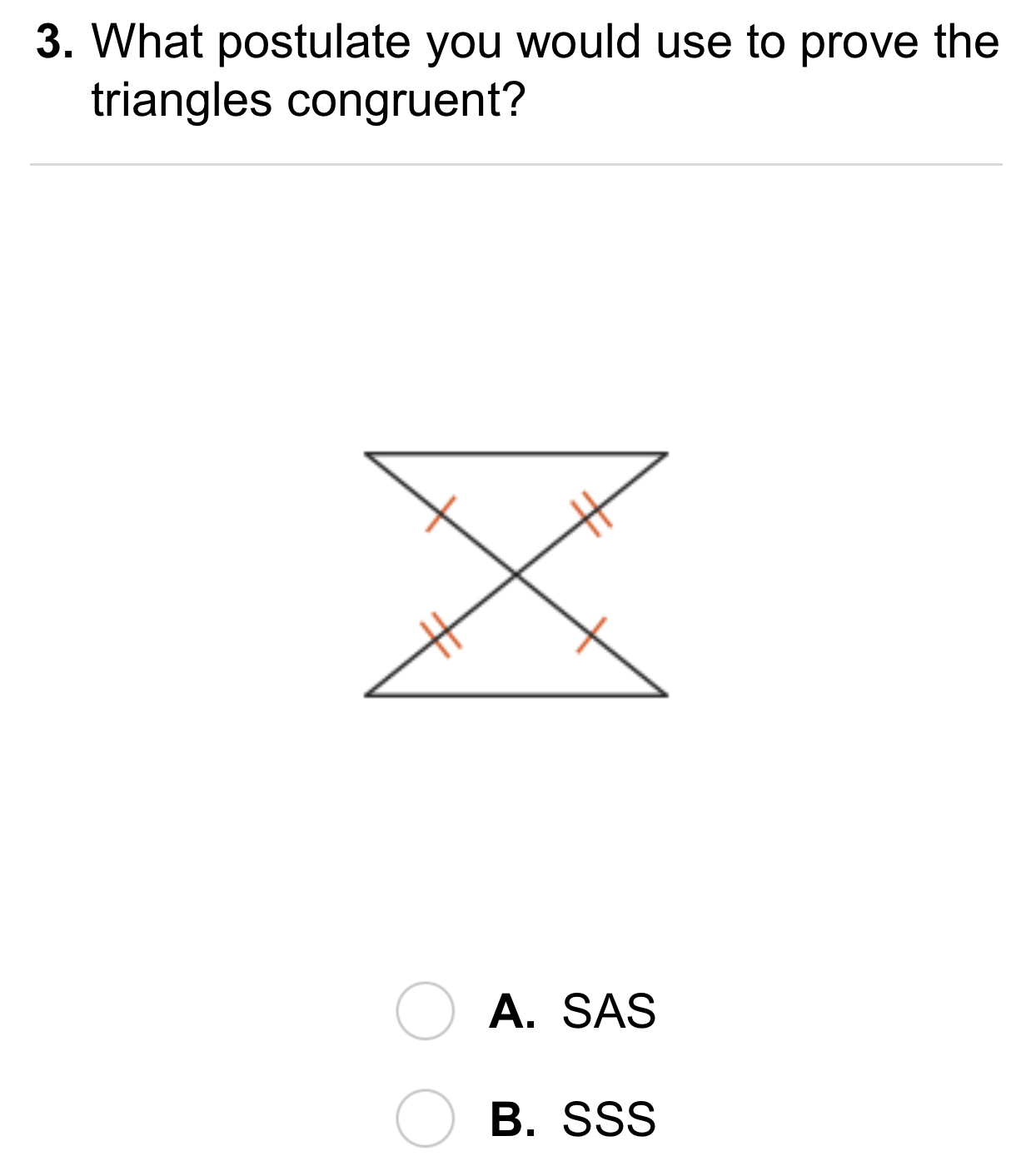10
10
10 pts
A
B11
11
10 pts
A
B12
10 pts
Compare and Contrast: How are the SSS Postulate and the SAS Postulate alike? How are they different?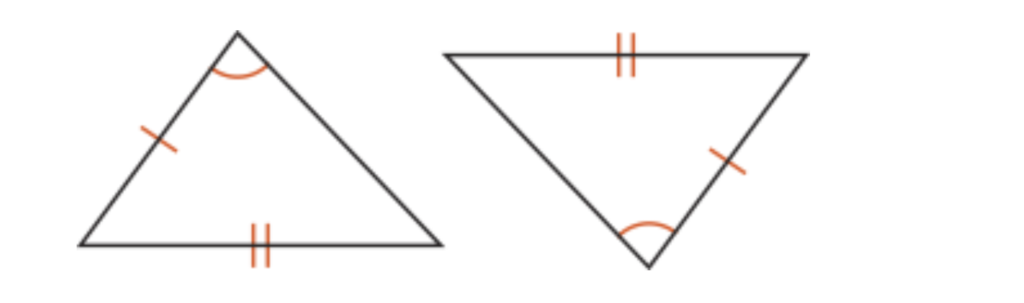13
14
10 pts
Reasoning: A carpenter trims a triangular peak of a house with three 7-ft pieces of molding. The carpenter uses 21 ft of molding to trim a second triangular peak. Are the two triangles formed congruent? Explain.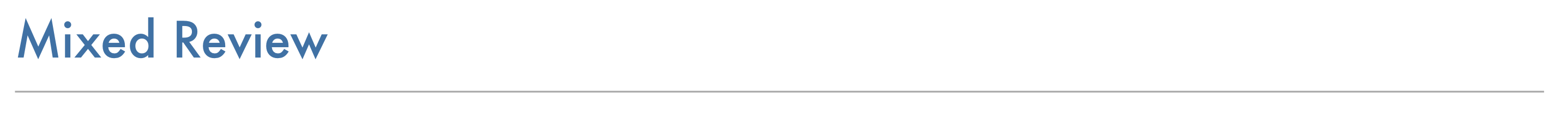15
10 pts
Review Lesson 4-1: Two quadrilaterals are congruent as described in the congruence statement below.
Categorize the items to match corresponding angles and sides.
• angle A
• segment EF
• segment BC
• angle G
• segment FG
• angle E
• segment AB
• angle C
16
10 pts
Review Lesson 2-2: Write the converse of the statement. Determine whether the statement and its converse are true or false. "If x = 3, then 2x = 6."
Converse: "If 2x = 6, then x = 3." Conditional: True. Converse: False.
Converse: "If x ≠ 3, then 2x ≠ 3." Conditional: True. Converse: False.
Converse: "If 2x = 6, then x = 3." Conditional: True. Converse: True.
Converse: "If x ≠ 3, then 2x ≠ 3." Conditional: True. Converse: True.
17
10 pts
Review Lesson 2-2: Is the converse of the statement true or false? "If x = 3, then x² = 9."
True
False
18
5 pts
Review Lesson 4-2: In △JHK, name the side that is included between ∠J and ∠H.
side JH
side KH
side KJ
19
5 pts
Review Lesson 4-2: In △NLM, name the angle that is included between side NM and side LN.
∠N
∠L
∠M20
20
10 pts
A
B
C
D
21
10 pts
Reflection: Math Success
Add to my formatives list

Formative uses cookies to allow us to better understand how the site is used. By continuing to use this site, you consent to the Terms of Service and Privacy Policy.